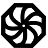# Programming-IdiomsImplementation
Rust

Be concise.

Be useful.

All contributions dictatorially edited by webmasters to match personal tastes.

Please try to avoid dependencies to third-party libraries and frameworks.

Implementation edit is for fixing errors and enhancing with metadata.

Instead of changing the code of the snippet, consider creating another Rust implementation.

Other implementations
`import "os"`
`dir, err := os.Getwd()`
`import std.path;`
`string dir = absolutePath;`
`dir := expandfilename('.');`
`import System.Directory`
`dir <- getCurrentDirectory`
`with Ada.Directories; use Ada.Directories;`
`Dir : String := Current_Directory;`
`use Cwd;`
`my \$dir = getcwd();`
`String path = this.getClass().getClassLoader().getResource("").getPath();`
`\$dir = getcwd();`
`dir = Dir.pwd`
`import os`
`dir = os.getcwd()`
`let dir = process.cwd ()`
`#include <unistd.h>`
`char *dir = getcwd(NULL, 0);`
`using System.IO;`
`string path = Directory.GetCurrentDirectory();`
`os.execute(cd)`
`#include <filesystem>`
`dir = std::filesystem::current_path();`